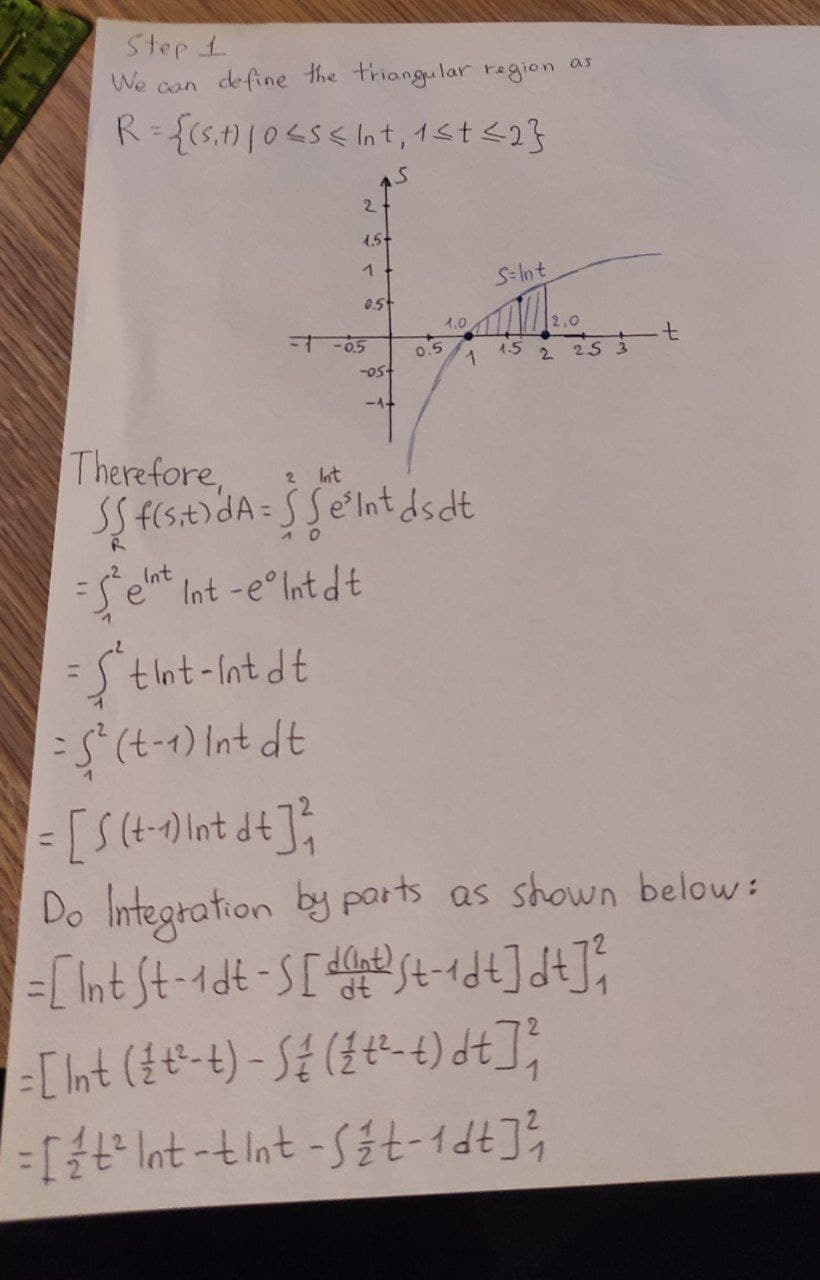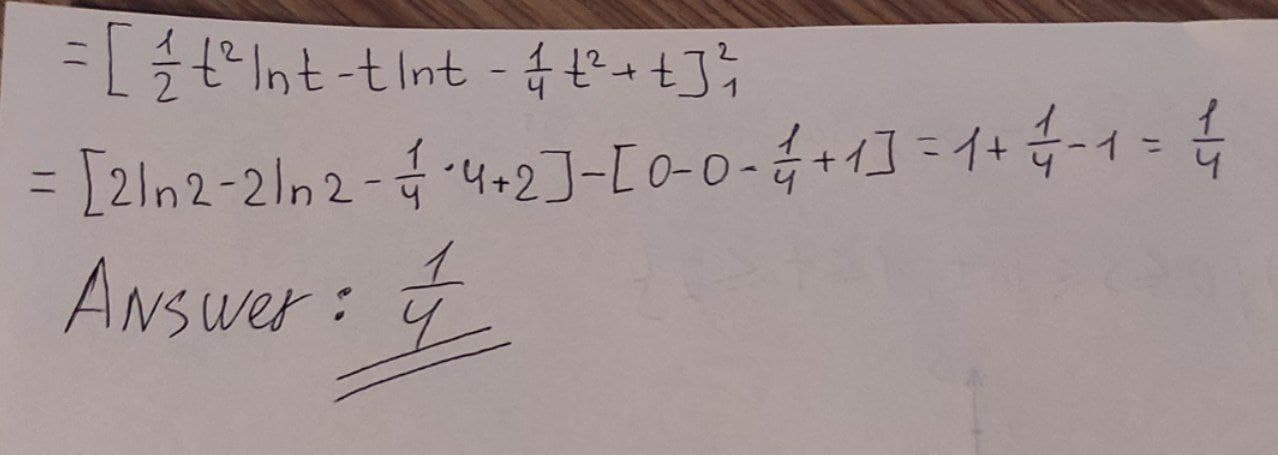# integrate f over the given region f(s,t)=e^sln t over the region in the first quadrant of the st-plane that lies above the curve s=ln t from t=1 to t=2

integrate f over the given region f(s,t)=e^sln t over the region in the first quadrant of the st-plane that lies above the curve s=ln t from t=1 to t=2
You can still ask an expert for help

• Questions are typically answered in as fast as 30 minutes

Solve your problem for the price of one coffee

• Math expert for every subject
• Pay only if we can solve itnick1337

See my phtoto for the right answer!